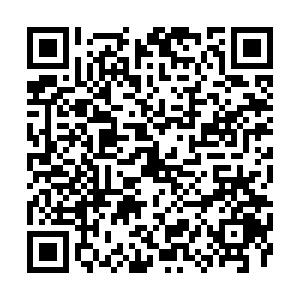## 留言板引用本文: 刘名生, 黄蕥媛. 关于k-折对称点的近于凸函数和拟凸函数子类的邻域[J]. 华南师范大学学报（自然科学版）, 2012, 44(1).On the neighborhoods of certain subclasses of close-to-convex and quasi-convex[J]. Journal of South China normal University (Natural Science Edition), 2012, 44(1).
 Citation: On the neighborhoods of certain subclasses of close-to-convex and quasi-convex[J]. Journal of South China normal University (Natural Science Edition), 2012, 44(1).• 中图分类号:

O 174.51

## On the neighborhoods of certain subclasses of close-to-convex and quasi-convex

• 摘要: 研究了关于$k$-折对称点的近于凸函数和拟凸函数子类的邻域。对于 ${\mathcal S_{s,\ n}^{(k)}}[A, B]$ 或者 ${\mathcal C_{s,\ n}^{(k)}}[A, B]$中的函数$f$, 得到了使得所有函数$g\in{\mathcal N_{\delta}}(f)$包含在 ${\mathcal S_{s,\ n}^{(k)}}[A, B]$内的充分条件，且 $\delta$ 是最好的可能。
•点击查看大图
##### 计量
• 文章访问数:  1206
• HTML全文浏览量:  88
• PDF下载量:  288
• 被引次数: 0
##### 出版历程
• 收稿日期:  2011-02-21
• 修回日期:  2011-05-04
• 刊出日期:  2012-02-25

### 目录/下载:  全尺寸图片 幻灯片
• 分享
• 用微信扫码二维码

分享至好友和朋友圈